## Design

### Concepts

#### Null Policy Classes

Associative containers are typically parametrized by various policies. For example, a hash-based associative container is parametrized by a hash-functor, transforming each key into an non-negative numerical type. Each such value is then further mapped into a position within the table. The mapping of a key into a position within the table is therefore a two-step process.

In some cases, instantiations are redundant. For example, when the keys are integers, it is possible to use a redundant hash policy, which transforms each key into its value.

In some other cases, these policies are irrelevant. For example, a hash-based associative container might transform keys into positions within a table by a different method than the two-step method described above. In such a case, the hash functor is simply irrelevant.

When a policy is either redundant or irrelevant, it can be replaced by `null_type`.

For example, a set is an associative container with one of its template parameters (the one for the mapped type) replaced with `null_type`. Other places simplifications are made possible with this technique include node updates in tree and trie data structures, and hash and probe functions for hash data structures.

#### Map and Set Semantics

##### Distinguishing Between Maps and Sets

Anyone familiar with the standard knows that there are four kinds of associative containers: maps, sets, multimaps, and multisets. The map datatype associates each key to some data.

Sets are associative containers that simply store keys - they do not map them to anything. In the standard, each map class has a corresponding set class. E.g., `std::map<int, char>` maps each `int` to a `char`, but `std::set<int, char>` simply stores `int`s. In this library, however, there are no distinct classes for maps and sets. Instead, an associative container's `Mapped` template parameter is a policy: if it is instantiated by `null_type`, then it is a "set"; otherwise, it is a "map". E.g.,

```	  cc_hash_table<int, char>
```

is a "map" mapping each int value to a char, but

```	  cc_hash_table<int, null_type>
```

is a type that uniquely stores int values.

Once the `Mapped` template parameter is instantiated by `null_type`, then the "set" acts very similarly to the standard's sets - it does not map each key to a distinct `null_type` object. Also, , the container's value_type is essentially its key_type - just as with the standard's sets .

The standard's multimaps and multisets allow, respectively, non-uniquely mapping keys and non-uniquely storing keys. As discussed, the reasons why this might be necessary are 1) that a key might be decomposed into a primary key and a secondary key, 2) that a key might appear more than once, or 3) any arbitrary combination of 1)s and 2)s. Correspondingly, one should use 1) "maps" mapping primary keys to secondary keys, 2) "maps" mapping keys to size types, or 3) any arbitrary combination of 1)s and 2)s. Thus, for example, an `std::multiset<int>` might be used to store multiple instances of integers, but using this library's containers, one might use

```	  tree<int, size_t>
```

i.e., a `map` of ints to size_ts.

These "multimaps" and "multisets" might be confusing to anyone familiar with the standard's `std::multimap` and `std::multiset`, because there is no clear correspondence between the two. For example, in some cases where one uses `std::multiset` in the standard, one might use in this library a "multimap" of "multisets" - i.e., a container that maps primary keys each to an associative container that maps each secondary key to the number of times it occurs.

When one uses a "multimap," one should choose with care the type of container used for secondary keys.

##### Alternatives to `std::multiset` and `std::multimap`

Brace onself: this library does not contain containers like `std::multimap` or `std::multiset`. Instead, these data structures can be synthesized via manipulation of the `Mapped` template parameter.

One maps the unique part of a key - the primary key, into an associative-container of the (originally) non-unique parts of the key - the secondary key. A primary associative-container is an associative container of primary keys; a secondary associative-container is an associative container of secondary keys.

Stepping back a bit, and starting in from the beginning.

Maps (or sets) allow mapping (or storing) unique-key values. The standard library also supplies associative containers which map (or store) multiple values with equivalent keys: `std::multimap`, `std::multiset`, `std::tr1::unordered_multimap`, and `unordered_multiset`. We first discuss how these might be used, then why we think it is best to avoid them.

Suppose one builds a simple bank-account application that records for each client (identified by an `std::string`) and account-id (marked by an unsigned long) - the balance in the account (described by a float). Suppose further that ordering this information is not useful, so a hash-based container is preferable to a tree based container. Then one can use

```	  std::tr1::unordered_map<std::pair<std::string, unsigned long>, float, ...>
```

which hashes every combination of client and account-id. This might work well, except for the fact that it is now impossible to efficiently list all of the accounts of a specific client (this would practically require iterating over all entries). Instead, one can use

```	  std::tr1::unordered_multimap<std::pair<std::string, unsigned long>, float, ...>
```

which hashes every client, and decides equivalence based on client only. This will ensure that all accounts belonging to a specific user are stored consecutively.

Also, suppose one wants an integers' priority queue (a container that supports `push`, `pop`, and `top` operations, the last of which returns the largest int) that also supports operations such as `find` and `lower_bound`. A reasonable solution is to build an adapter over `std::set<int>`. In this adapter, `push` will just call the tree-based associative container's `insert` method; `pop` will call its `end` method, and use it to return the preceding element (which must be the largest). Then this might work well, except that the container object cannot hold multiple instances of the same integer (`push(4)`, will be a no-op if `4` is already in the container object). If multiple keys are necessary, then one might build the adapter over an `std::multiset<int>`.

The standard library's non-unique-mapping containers are useful when (1) a key can be decomposed in to a primary key and a secondary key, (2) a key is needed multiple times, or (3) any combination of (1) and (2).

The graphic below shows how the standard library's container design works internally; in this figure nodes shaded equally represent equivalent-key values. Equivalent keys are stored consecutively using the properties of the underlying data structure: binary search trees (label A) store equivalent-key values consecutively (in the sense of an in-order walk) naturally; collision-chaining hash tables (label B) store equivalent-key values in the same bucket, the bucket can be arranged so that equivalent-key values are consecutive.

Figure 22.8. Non-unique Mapping Standard Containers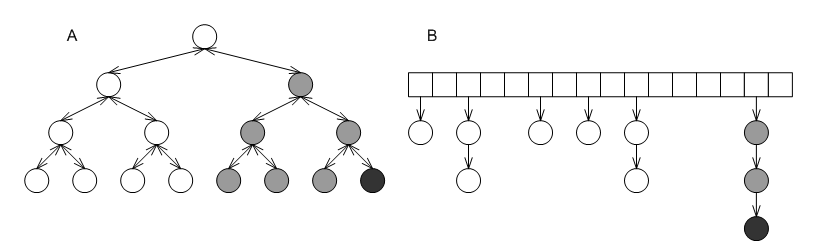Put differently, the standards' non-unique mapping associative-containers are associative containers that map primary keys to linked lists that are embedded into the container. The graphic below shows again the two containers from the first graphic above, this time with the embedded linked lists of the grayed nodes marked explicitly.

Figure 22.9.  Effect of embedded lists in `std::multimap`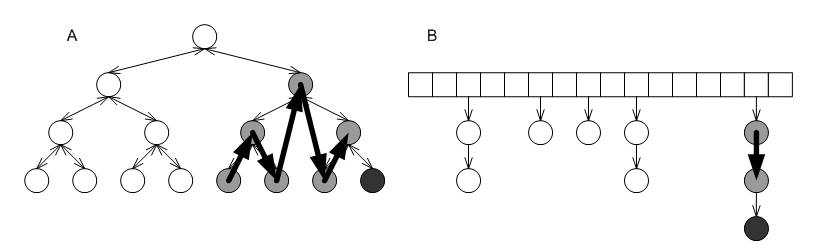1. The underlying data structure embeds the linked lists according to its own consideration, which means that the search path for a value might include several different equivalent-key values. For example, the search path for the the black node in either of the first graphic, labels A or B, includes more than a single gray node.

2. The links of the linked lists are the underlying data structures' nodes, which typically are quite structured. In the case of tree-based containers (the grapic above, label B), each "link" is actually a node with three pointers (one to a parent and two to children), and a relatively-complicated iteration algorithm. The linked lists, therefore, can take up quite a lot of memory, and iterating over all values equal to a given key (through the return value of the standard library's `equal_range`) can be expensive.

3. The primary key is stored multiply; this uses more memory.

4. Finally, the interface of this design excludes several useful underlying data structures. Of all the unordered self-organizing data structures, practically only collision-chaining hash tables can (efficiently) guarantee that equivalent-key values are stored consecutively.

The above reasons hold even when the ratio of secondary keys to primary keys (or average number of identical keys) is small, but when it is large, there are more severe problems:

1. The underlying data structures order the links inside each embedded linked-lists according to their internal considerations, which effectively means that each of the links is unordered. Irrespective of the underlying data structure, searching for a specific value can degrade to linear complexity.

2. Similarly to the above point, it is impossible to apply to the secondary keys considerations that apply to primary keys. For example, it is not possible to maintain secondary keys by sorted order.

3. While the interface "understands" that all equivalent-key values constitute a distinct list (through `equal_range`), the underlying data structure typically does not. This means that operations such as erasing from a tree-based container all values whose keys are equivalent to a a given key can be super-linear in the size of the tree; this is also true also for several other operations that target a specific list.

In this library, all associative containers map (or store) unique-key values. One can (1) map primary keys to secondary associative-containers (containers of secondary keys) or non-associative containers (2) map identical keys to a size-type representing the number of times they occur, or (3) any combination of (1) and (2). Instead of allowing multiple equivalent-key values, this library supplies associative containers based on underlying data structures that are suitable as secondary associative-containers.

In the figure below, labels A and B show the equivalent underlying data structures in this library, as mapped to the first graphic above. Labels A and B, respectively. Each shaded box represents some size-type or secondary associative-container.

Figure 22.10. Non-unique Mapping Containers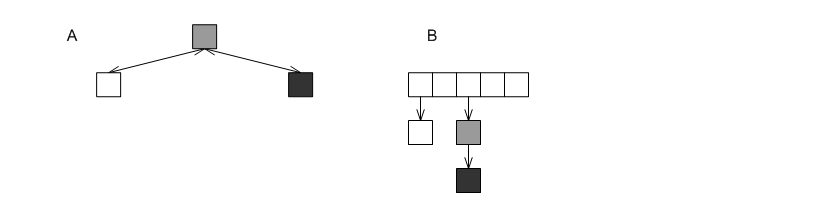In the first example above, then, one would use an associative container mapping each user to an associative container which maps each application id to a start time (see `example/basic_multimap.cc`); in the second example, one would use an associative container mapping each `int` to some size-type indicating the number of times it logically occurs (see `example/basic_multiset.cc`.

See the discussion in list-based container types for containers especially suited as secondary associative-containers.

#### Iterator Semantics

##### Point and Range Iterators

Iterator concepts are bifurcated in this design, and are comprised of point-type and range-type iteration.

A point-type iterator is an iterator that refers to a specific element as returned through an associative-container's `find` method.

A range-type iterator is an iterator that is used to go over a sequence of elements, as returned by a container's `find` method.

A point-type method is a method that returns a point-type iterator; a range-type method is a method that returns a range-type iterator.

For most containers, these types are synonymous; for self-organizing containers, such as hash-based containers or priority queues, these are inherently different (in any implementation, including that of C++ standard library components), but in this design, it is made explicit. They are distinct types.

##### Distinguishing Point and Range Iterators

When using this library, is necessary to differentiate between two types of methods and iterators: point-type methods and iterators, and range-type methods and iterators. Each associative container's interface includes the methods:

```	  point_const_iterator
find(const_key_reference r_key) const;

point_iterator
find(const_key_reference r_key);

std::pair<point_iterator,bool>
insert(const_reference r_val);
```

The relationship between these iterator types varies between container types. The figure below shows the most general invariant between point-type and range-type iterators: In A `iterator`, can always be converted to `point_iterator`. In B shows invariants for order-preserving containers: point-type iterators are synonymous with range-type iterators. Orthogonally, Cshows invariants for "set" containers: iterators are synonymous with const iterators.

Figure 22.11. Point Iterator Hierarchy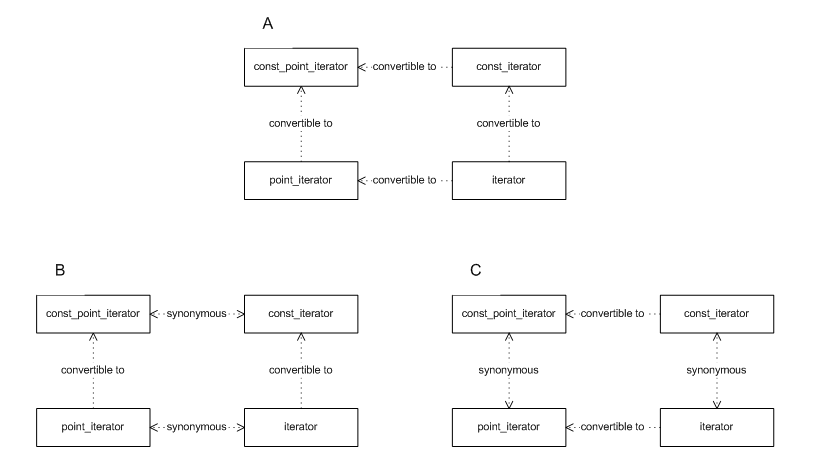Note that point-type iterators in self-organizing containers (hash-based associative containers) lack movement operators, such as `operator++` - in fact, this is the reason why this library differentiates from the standard C++ librarys design on this point.

Typically, one can determine an iterator's movement capabilities using `std::iterator_traits<It>iterator_category`, which is a `struct` indicating the iterator's movement capabilities. Unfortunately, none of the standard predefined categories reflect a pointer's not having any movement capabilities whatsoever. Consequently, `pb_ds` adds a type `trivial_iterator_tag` (whose name is taken from a concept in C++ standardese, which is the category of iterators with no movement capabilities.) All other standard C++ library tags, such as `forward_iterator_tag` retain their common use.

##### Invalidation Guarantees

If one manipulates a container object, then iterators previously obtained from it can be invalidated. In some cases a previously-obtained iterator cannot be de-referenced; in other cases, the iterator's next or previous element might have changed unpredictably. This corresponds exactly to the question whether a point-type or range-type iterator (see previous concept) is valid or not. In this design, one can query a container (in compile time) about its invalidation guarantees.

Given three different types of associative containers, a modifying operation (in that example, `erase`) invalidated iterators in three different ways: the iterator of one container remained completely valid - it could be de-referenced and incremented; the iterator of a different container could not even be de-referenced; the iterator of the third container could be de-referenced, but its "next" iterator changed unpredictably.

Distinguishing between find and range types allows fine-grained invalidation guarantees, because these questions correspond exactly to the question of whether point-type iterators and range-type iterators are valid. The graphic below shows tags corresponding to different types of invalidation guarantees.

Figure 22.12. Invalidation Guarantee Tags Hierarchy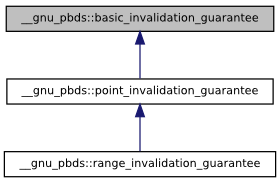• `basic_invalidation_guarantee` corresponds to a basic guarantee that a point-type iterator, a found pointer, or a found reference, remains valid as long as the container object is not modified.

• `point_invalidation_guarantee` corresponds to a guarantee that a point-type iterator, a found pointer, or a found reference, remains valid even if the container object is modified.

• `range_invalidation_guarantee` corresponds to a guarantee that a range-type iterator remains valid even if the container object is modified.

To find the invalidation guarantee of a container, one can use

```	  typename container_traits<Cntnr>::invalidation_guarantee
```

Note that this hierarchy corresponds to the logic it represents: if a container has range-invalidation guarantees, then it must also have find invalidation guarantees; correspondingly, its invalidation guarantee (in this case `range_invalidation_guarantee`) can be cast to its base class (in this case `point_invalidation_guarantee`). This means that this this hierarchy can be used easily using standard metaprogramming techniques, by specializing on the type of `invalidation_guarantee`.

These types of problems were addressed, in a more general setting, in [biblio.meyers96more] - Item 2. In our opinion, an invalidation-guarantee hierarchy would solve these problems in all container types - not just associative containers.

#### Genericity

The design attempts to address the following problem of data-structure genericity. When writing a function manipulating a generic container object, what is the behavior of the object? Suppose one writes

```	template<typename Cntnr>
void
some_op_sequence(Cntnr &r_container)
{
...
}
```

then one needs to address the following questions in the body of `some_op_sequence`:

• Which types and methods does `Cntnr` support? Containers based on hash tables can be queries for the hash-functor type and object; this is meaningless for tree-based containers. Containers based on trees can be split, joined, or can erase iterators and return the following iterator; this cannot be done by hash-based containers.

• What are the exception and invalidation guarantees of `Cntnr`? A container based on a probing hash-table invalidates all iterators when it is modified; this is not the case for containers based on node-based trees. Containers based on a node-based tree can be split or joined without exceptions; this is not the case for containers based on vector-based trees.

• How does the container maintain its elements? Tree-based and Trie-based containers store elements by key order; others, typically, do not. A container based on a splay trees or lists with update policies "cache" "frequently accessed" elements; containers based on most other underlying data structures do not.

• How does one query a container about characteristics and capabilities? What is the relationship between two different data structures, if anything?

The remainder of this section explains these issues in detail.

##### Tag

Tags are very useful for manipulating generic types. For example, if `It` is an iterator class, then ```typename It::iterator_category``` or ```typename std::iterator_traits<It>::iterator_category``` will yield its category, and ```typename std::iterator_traits<It>::value_type``` will yield its value type.

This library contains a container tag hierarchy corresponding to the diagram below.

Figure 22.13. Container Tag Hierarchy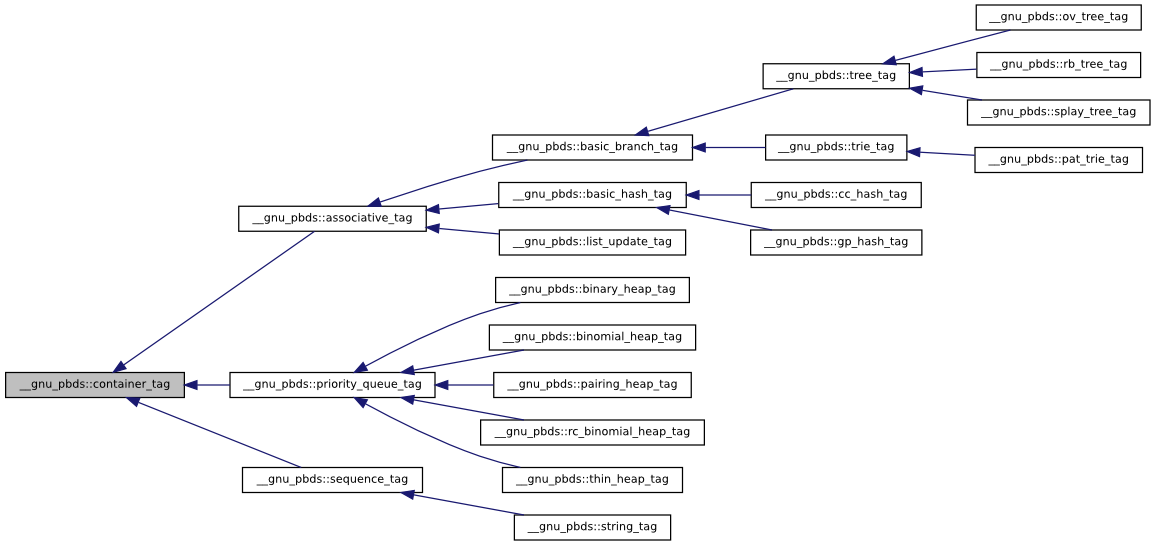Given any container Cntnr, the tag of the underlying data structure can be found via ```typename Cntnr::container_category```.

##### Traits

Additionally, a traits mechanism can be used to query a container type for its attributes. Given any container `Cntnr`, then `<Cntnr>` is a traits class identifying the properties of the container.

To find if a container can throw when a key is erased (which is true for vector-based trees, for example), one can use

`container_traits<Cntnr>::erase_can_throw`

Some of the definitions in `container_traits` are dependent on other definitions. If `container_traits<Cntnr>::order_preserving` is `true` (which is the case for containers based on trees and tries), then the container can be split or joined; in this case, `container_traits<Cntnr>::split_join_can_throw` indicates whether splits or joins can throw exceptions (which is true for vector-based trees); otherwise `container_traits<Cntnr>::split_join_can_throw` will yield a compilation error. (This is somewhat similar to a compile-time version of the COM model).

### By Container

#### hash

##### Interface

The collision-chaining hash-based container has the following declaration.

```	  template<
typename Key,
typename Mapped,
typename Hash_Fn = std::hash<Key>,
typename Eq_Fn = std::equal_to<Key>,
typename Resize_Policy = default explained below.
bool Store_Hash = false,
typename Allocator = std::allocator<char> >
class cc_hash_table;
```

The parameters have the following meaning:

1. `Key` is the key type.

2. `Mapped` is the mapped-policy.

3. `Hash_Fn` is a key hashing functor.

4. `Eq_Fn` is a key equivalence functor.

5. `Comb_Hash_Fn` is a range-hashing_functor; it describes how to translate hash values into positions within the table.

6. `Resize_Policy` describes how a container object should change its internal size.

7. `Store_Hash` indicates whether the hash value should be stored with each entry.

8. `Allocator` is an allocator type.

The probing hash-based container has the following declaration.

```	  template<
typename Key,
typename Mapped,
typename Hash_Fn = std::hash<Key>,
typename Eq_Fn = std::equal_to<Key>,
typename Probe_Fn = default explained below.
typename Resize_Policy = default explained below.
bool Store_Hash = false,
typename Allocator =  std::allocator<char> >
class gp_hash_table;
```

The parameters are identical to those of the collision-chaining container, except for the following.

1. `Comb_Probe_Fn` describes how to transform a probe sequence into a sequence of positions within the table.

2. `Probe_Fn` describes a probe sequence policy.

Some of the default template values depend on the values of other parameters, and are explained below.

##### Details
###### General

Following is an explanation of some functions which hashing involves. The graphic below illustrates the discussion.

Figure 22.14. Hash functions, ranged-hash functions, and range-hashing functions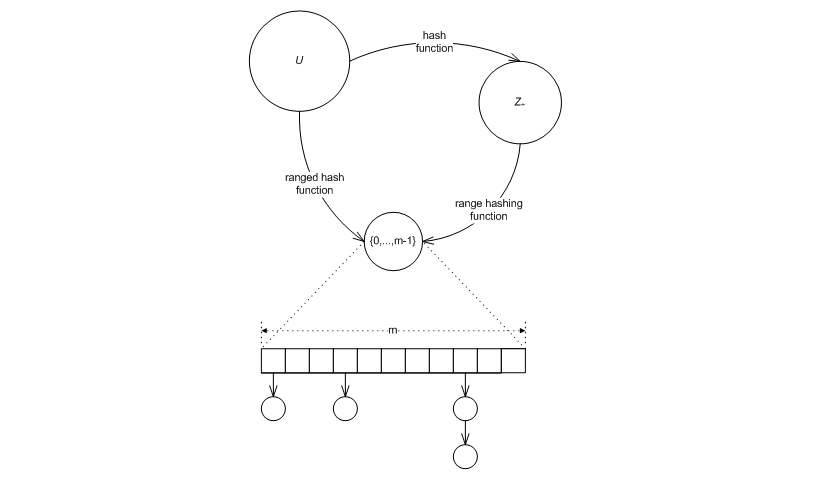Let U be a domain (e.g., the integers, or the strings of 3 characters). A hash-table algorithm needs to map elements of U "uniformly" into the range [0,..., m - 1] (where m is a non-negative integral value, and is, in general, time varying). I.e., the algorithm needs a ranged-hash function

f : U × Z+ → Z+

such that for any u in U ,

0 ≤ f(u, m) ≤ m - 1

and which has "good uniformity" properties (say [biblio.knuth98sorting].) One common solution is to use the composition of the hash function

h : U → Z+ ,

which maps elements of U into the non-negative integrals, and

g : Z+ × Z+ → Z+,

which maps a non-negative hash value, and a non-negative range upper-bound into a non-negative integral in the range between 0 (inclusive) and the range upper bound (exclusive), i.e., for any r in Z+,

0 ≤ g(r, m) ≤ m - 1

The resulting ranged-hash function, is

Equation 22.1. Ranged Hash Function

f(u , m) = g(h(u), m)

From the above, it is obvious that given g and h, f can always be composed (however the converse is not true). The standard's hash-based containers allow specifying a hash function, and use a hard-wired range-hashing function; the ranged-hash function is implicitly composed.

The above describes the case where a key is to be mapped into a single position within a hash table, e.g., in a collision-chaining table. In other cases, a key is to be mapped into a sequence of positions within a table, e.g., in a probing table. Similar terms apply in this case: the table requires a ranged probe function, mapping a key into a sequence of positions withing the table. This is typically achieved by composing a hash function mapping the key into a non-negative integral type, a probe function transforming the hash value into a sequence of hash values, and a range-hashing function transforming the sequence of hash values into a sequence of positions.

###### Range Hashing

Some common choices for range-hashing functions are the division, multiplication, and middle-square methods ([biblio.knuth98sorting]), defined as

Equation 22.2. Range-Hashing, Division Method

g(r, m) = r mod m

g(r, m) = ⌈ u/v ( a r mod v ) ⌉

and

g(r, m) = ⌈ u/v ( r2 mod v ) ⌉

respectively, for some positive integrals u and v (typically powers of 2), and some a. Each of these range-hashing functions works best for some different setting.

The division method (see above) is a very common choice. However, even this single method can be implemented in two very different ways. It is possible to implement using the low level % (modulo) operation (for any m), or the low level & (bit-mask) operation (for the case where m is a power of 2), i.e.,

Equation 22.3. Division via Prime Modulo

g(r, m) = r % m

and

Equation 22.4. Division via Bit Mask

g(r, m) = r & m - 1, (with m = 2k for some k)

respectively.

The % (modulo) implementation has the advantage that for m a prime far from a power of 2, g(r, m) is affected by all the bits of r (minimizing the chance of collision). It has the disadvantage of using the costly modulo operation. This method is hard-wired into SGI's implementation .

The & (bit-mask) implementation has the advantage of relying on the fast bit-wise and operation. It has the disadvantage that for g(r, m) is affected only by the low order bits of r. This method is hard-wired into Dinkumware's implementation.

###### Ranged Hash

In cases it is beneficial to allow the client to directly specify a ranged-hash hash function. It is true, that the writer of the ranged-hash function cannot rely on the values of m having specific numerical properties suitable for hashing (in the sense used in [biblio.knuth98sorting]), since the values of m are determined by a resize policy with possibly orthogonal considerations.

There are two cases where a ranged-hash function can be superior. The firs is when using perfect hashing: the second is when the values of m can be used to estimate the "general" number of distinct values required. This is described in the following.

Let

s = [ s0,..., st - 1]

be a string of t characters, each of which is from domain S. Consider the following ranged-hash function:

Equation 22.5.  A Standard String Hash Function

f1(s, m) = ∑ i = 0t - 1 si ai mod m

where a is some non-negative integral value. This is the standard string-hashing function used in SGI's implementation (with a = 5). Its advantage is that it takes into account all of the characters of the string.

Now assume that s is the string representation of a of a long DNA sequence (and so S = {'A', 'C', 'G', 'T'}). In this case, scanning the entire string might be prohibitively expensive. A possible alternative might be to use only the first k characters of the string, where

|S|k ≥ m ,

i.e., using the hash function

Equation 22.6.  Only k String DNA Hash

f2(s, m) = ∑ i = 0k - 1 si ai mod m

requiring scanning over only

k = log4( m )

characters.

Other more elaborate hash-functions might scan k characters starting at a random position (determined at each resize), or scanning k random positions (determined at each resize), i.e., using

f3(s, m) = ∑ i = r0r0 + k - 1 si ai mod m ,

or

f4(s, m) = ∑ i = 0k - 1 sri ari mod m ,

respectively, for r0,..., rk-1 each in the (inclusive) range [0,...,t-1].

It should be noted that the above functions cannot be decomposed as per a ranged hash composed of hash and range hashing.

###### Implementation

This sub-subsection describes the implementation of the above in this library. It first explains range-hashing functions in collision-chaining tables, then ranged-hash functions in collision-chaining tables, then probing-based tables, and finally lists the relevant classes in this library.

###### Range-Hashing and Ranged-Hashes in Collision-Chaining Tables

`cc_hash_table` is parametrized by `Hash_Fn` and `Comb_Hash_Fn`, a hash functor and a combining hash functor, respectively.

In general, `Comb_Hash_Fn` is considered a range-hashing functor. `cc_hash_table` synthesizes a ranged-hash function from `Hash_Fn` and `Comb_Hash_Fn`. The figure below shows an `insert` sequence diagram for this case. The user inserts an element (point A), the container transforms the key into a non-negative integral using the hash functor (points B and C), and transforms the result into a position using the combining functor (points D and E).

Figure 22.15. Insert hash sequence diagram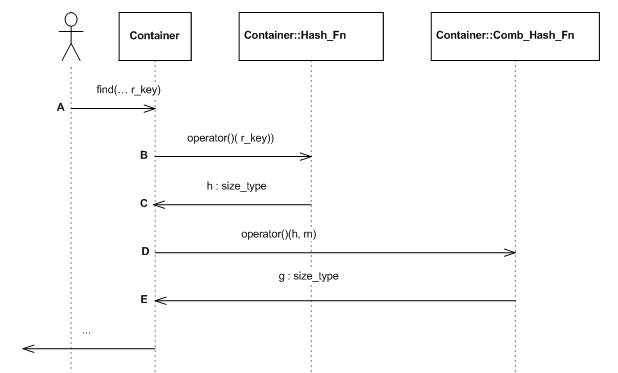If `cc_hash_table`'s hash-functor, `Hash_Fn` is instantiated by `null_type` , then `Comb_Hash_Fn` is taken to be a ranged-hash function. The graphic below shows an `insert` sequence diagram. The user inserts an element (point A), the container transforms the key into a position using the combining functor (points B and C).

Figure 22.16. Insert hash sequence diagram with a null policy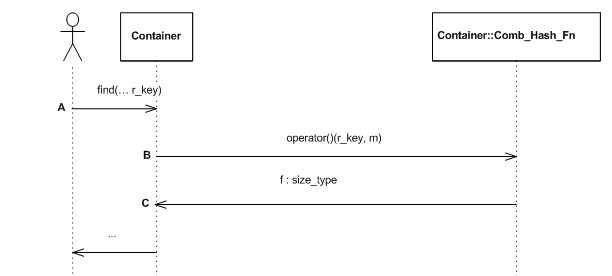###### Probing tables

`gp_hash_table` is parametrized by `Hash_Fn`, `Probe_Fn`, and `Comb_Probe_Fn`. As before, if `Hash_Fn` and `Probe_Fn` are both `null_type`, then `Comb_Probe_Fn` is a ranged-probe functor. Otherwise, `Hash_Fn` is a hash functor, `Probe_Fn` is a functor for offsets from a hash value, and `Comb_Probe_Fn` transforms a probe sequence into a sequence of positions within the table.

###### Pre-Defined Policies

This library contains some pre-defined classes implementing range-hashing and probing functions:

1. `direct_mask_range_hashing` and `direct_mod_range_hashing` are range-hashing functions based on a bit-mask and a modulo operation, respectively.

2. `linear_probe_fn`, and `quadratic_probe_fn` are a linear probe and a quadratic probe function, respectively.

The graphic below shows the relationships.

Figure 22.17. Hash policy class diagram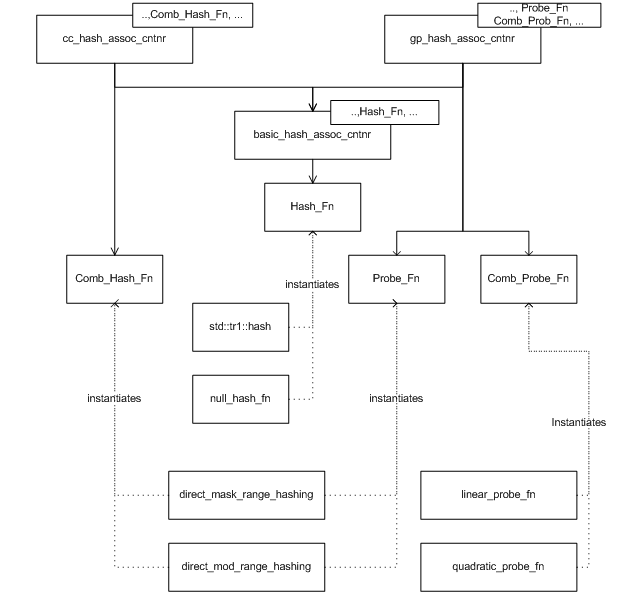###### General

Hash-tables, as opposed to trees, do not naturally grow or shrink. It is necessary to specify policies to determine how and when a hash table should change its size. Usually, resize policies can be decomposed into orthogonal policies:

1. A size policy indicating how a hash table should grow (e.g., it should multiply by powers of 2).

2. A trigger policy indicating when a hash table should grow (e.g., a load factor is exceeded).

###### Size Policies

Size policies determine how a hash table changes size. These policies are simple, and there are relatively few sensible options. An exponential-size policy (with the initial size and growth factors both powers of 2) works well with a mask-based range-hashing function, and is the hard-wired policy used by Dinkumware. A prime-list based policy works well with a modulo-prime range hashing function and is the hard-wired policy used by SGI's implementation.

###### Trigger Policies

Trigger policies determine when a hash table changes size. Following is a description of two policies: load-check policies, and collision-check policies.

Load-check policies are straightforward. The user specifies two factors, Αmin and Αmax, and the hash table maintains the invariant that

Αmin ≤ (number of stored elements) / (hash-table size) ≤ Αmaxload factor min max

Collision-check policies work in the opposite direction of load-check policies. They focus on keeping the number of collisions moderate and hoping that the size of the table will not grow very large, instead of keeping a moderate load-factor and hoping that the number of collisions will be small. A maximal collision-check policy resizes when the longest probe-sequence grows too large.

Consider the graphic below. Let the size of the hash table be denoted by m, the length of a probe sequence be denoted by k, and some load factor be denoted by Α. We would like to calculate the minimal length of k, such that if there were Α m elements in the hash table, a probe sequence of length k would be found with probability at most 1/m.

Figure 22.18. Balls and bins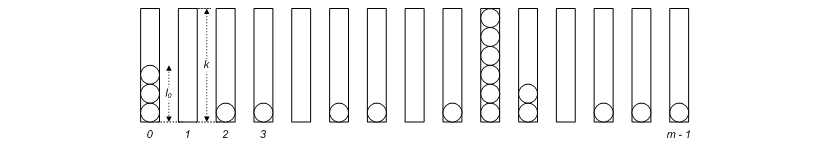Denote the probability that a probe sequence of length k appears in bin i by pi, the length of the probe sequence of bin i by li, and assume uniform distribution. Then

Equation 22.7.  Probability of Probe Sequence of Length k

p1 =

P(l1 ≥ k) =

P(l1 ≥ α ( 1 + k / α - 1) ≤ (a)

e ^ ( - ( α ( k / α - 1 )2 ) /2)

where (a) follows from the Chernoff bound ([biblio.motwani95random]). To calculate the probability that some bin contains a probe sequence greater than k, we note that the li are negatively-dependent ([biblio.dubhashi98neg]) . Let I(.) denote the indicator function. Then

Equation 22.8.  Probability Probe Sequence in Some Bin

P( existsi li ≥ k ) =

P ( ∑ i = 1m I(li ≥ k) ≥ 1 ) =

P ( ∑ i = 1m I ( li ≥ k ) ≥ m p1 ( 1 + 1 / (m p1) - 1 ) ) ≤ (a)

e ^ ( ( - m p1 ( 1 / (m p1) - 1 ) 2 ) / 2 ) ,

where (a) follows from the fact that the Chernoff bound can be applied to negatively-dependent variables ([biblio.dubhashi98neg]). Inserting the first probability equation into the second one, and equating with 1/m, we obtain

k ~ √ ( 2 α ln 2 m ln(m) ) ) .

###### Implementation

This sub-subsection describes the implementation of the above in this library. It first describes resize policies and their decomposition into trigger and size policies, then describes pre-defined classes, and finally discusses controlled access the policies' internals.

###### Decomposition

Each hash-based container is parametrized by a `Resize_Policy` parameter; the container derives `public`ly from `Resize_Policy`. For example:

```		cc_hash_table<typename Key,
typename Mapped,
...
typename Resize_Policy
...> : public Resize_Policy
```

As a container object is modified, it continuously notifies its `Resize_Policy` base of internal changes (e.g., collisions encountered and elements being inserted). It queries its `Resize_Policy` base whether it needs to be resized, and if so, to what size.

The graphic below shows a (possible) sequence diagram of an insert operation. The user inserts an element; the hash table notifies its resize policy that a search has started (point A); in this case, a single collision is encountered - the table notifies its resize policy of this (point B); the container finally notifies its resize policy that the search has ended (point C); it then queries its resize policy whether a resize is needed, and if so, what is the new size (points D to G); following the resize, it notifies the policy that a resize has completed (point H); finally, the element is inserted, and the policy notified (point I).

Figure 22.19. Insert resize sequence diagram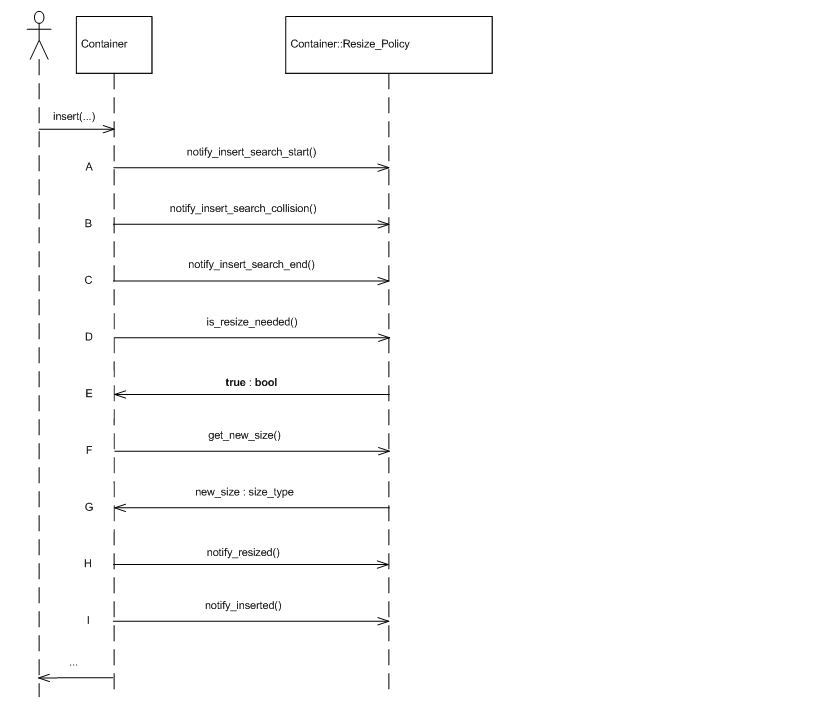In practice, a resize policy can be usually orthogonally decomposed to a size policy and a trigger policy. Consequently, the library contains a single class for instantiating a resize policy: `hash_standard_resize_policy` is parametrized by `Size_Policy` and `Trigger_Policy`, derives `public`ly from both, and acts as a standard delegate ([biblio.gof]) to these policies.

The two graphics immediately below show sequence diagrams illustrating the interaction between the standard resize policy and its trigger and size policies, respectively.

Figure 22.20. Standard resize policy trigger sequence diagram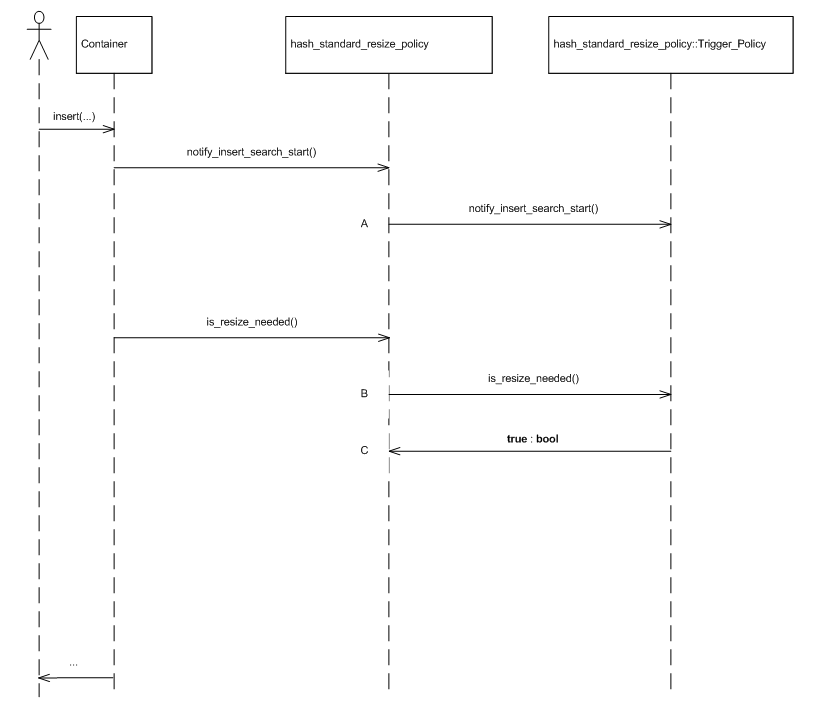Figure 22.21. Standard resize policy size sequence diagram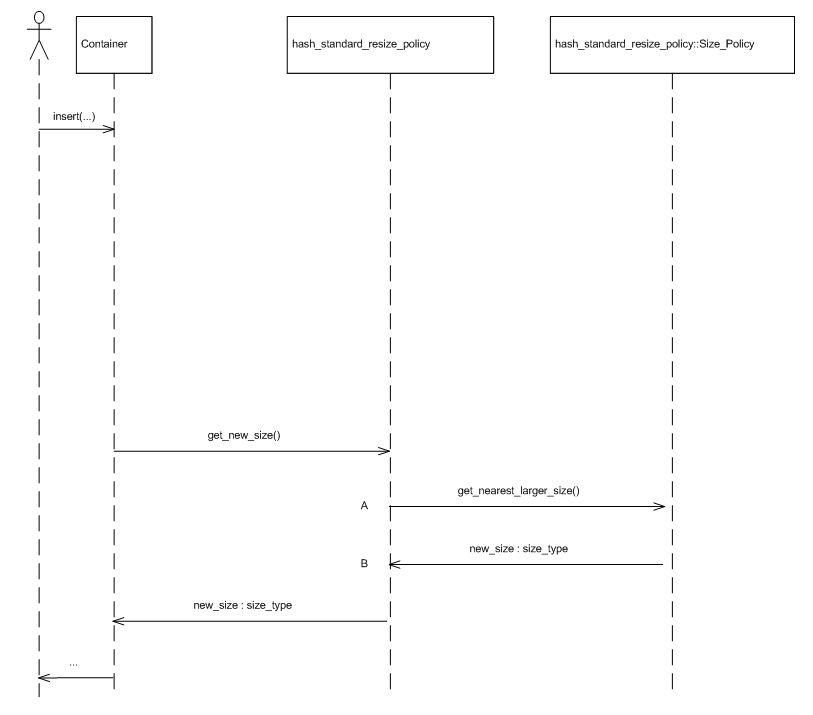###### Predefined Policies

The library includes the following instantiations of size and trigger policies:

1. `hash_load_check_resize_trigger` implements a load check trigger policy.

2. `cc_hash_max_collision_check_resize_trigger` implements a collision check trigger policy.

3. `hash_exponential_size_policy` implements an exponential-size policy (which should be used with mask range hashing).

4. `hash_prime_size_policy` implementing a size policy based on a sequence of primes (which should be used with mod range hashing

The graphic below gives an overall picture of the resize-related classes. `basic_hash_table` is parametrized by `Resize_Policy`, which it subclasses publicly. This class is currently instantiated only by `hash_standard_resize_policy`. `hash_standard_resize_policy` itself is parametrized by `Trigger_Policy` and `Size_Policy`. Currently, `Trigger_Policy` is instantiated by `hash_load_check_resize_trigger`, or `cc_hash_max_collision_check_resize_trigger`; `Size_Policy` is instantiated by `hash_exponential_size_policy`, or `hash_prime_size_policy`.

There are cases where (controlled) access to resize policies' internals is beneficial. E.g., it is sometimes useful to query a hash-table for the table's actual size (as opposed to its `size()` - the number of values it currently holds); it is sometimes useful to set a table's initial size, externally resize it, or change load factors.

Clearly, supporting such methods both decreases the encapsulation of hash-based containers, and increases the diversity between different associative-containers' interfaces. Conversely, omitting such methods can decrease containers' flexibility.

In order to avoid, to the extent possible, the above conflict, the hash-based containers themselves do not address any of these questions; this is deferred to the resize policies, which are easier to change or replace. Thus, for example, neither `cc_hash_table` nor `gp_hash_table` contain methods for querying the actual size of the table; this is deferred to `hash_standard_resize_policy`.

Furthermore, the policies themselves are parametrized by template arguments that determine the methods they support ( [biblio.alexandrescu01modern] shows techniques for doing so). `hash_standard_resize_policy` is parametrized by `External_Size_Access` that determines whether it supports methods for querying the actual size of the table or resizing it. `hash_load_check_resize_trigger` is parametrized by `External_Load_Access` that determines whether it supports methods for querying or modifying the loads. `cc_hash_max_collision_check_resize_trigger` is parametrized by `External_Load_Access` that determines whether it supports methods for querying the load.

Some operations, for example, resizing a container at run time, or changing the load factors of a load-check trigger policy, require the container itself to resize. As mentioned above, the hash-based containers themselves do not contain these types of methods, only their resize policies. Consequently, there must be some mechanism for a resize policy to manipulate the hash-based container. As the hash-based container is a subclass of the resize policy, this is done through virtual methods. Each hash-based container has a `private` `virtual` method:

```		virtual void
do_resize
(size_type new_size);
```

which resizes the container. Implementations of `Resize_Policy` can export public methods for resizing the container externally; these methods internally call `do_resize` to resize the table.

###### Policy Interactions

Hash-tables are unfortunately especially susceptible to choice of policies. One of the more complicated aspects of this is that poor combinations of good policies can form a poor container. Following are some considerations.

###### probe/size/trigger

Some combinations do not work well for probing containers. For example, combining a quadratic probe policy with an exponential size policy can yield a poor container: when an element is inserted, a trigger policy might decide that there is no need to resize, as the table still contains unused entries; the probe sequence, however, might never reach any of the unused entries.

Unfortunately, this library cannot detect such problems at compilation (they are halting reducible). It therefore defines an exception class `insert_error` to throw an exception in this case.

###### hash/trigger

Some trigger policies are especially susceptible to poor hash functions. Suppose, as an extreme case, that the hash function transforms each key to the same hash value. After some inserts, a collision detecting policy will always indicate that the container needs to grow.

The library, therefore, by design, limits each operation to one resize. For each `insert`, for example, it queries only once whether a resize is needed.

###### equivalence functors/storing hash values/hash

`cc_hash_table` and `gp_hash_table` are parametrized by an equivalence functor and by a `Store_Hash` parameter. If the latter parameter is `true`, then the container stores with each entry a hash value, and uses this value in case of collisions to determine whether to apply a hash value. This can lower the cost of collision for some types, but increase the cost of collisions for other types.

If a ranged-hash function or ranged probe function is directly supplied, however, then it makes no sense to store the hash value with each entry. This library's container will fail at compilation, by design, if this is attempted.

Assume a size policy issues an increasing sequence of sizes a, a q, a q1, a q2, ... For example, an exponential size policy might issue the sequence of sizes 8, 16, 32, 64, ...

If a load-check trigger policy is used, with loads αmin and αmax, respectively, then it is a good idea to have:

1. αmax ~ 1 / q

2. αmin < 1 / (2 q)

This will ensure that the amortized hash cost of each modifying operation is at most approximately 3.

αmin ~ αmax is, in any case, a bad choice, and αmin > α max is horrendous.

#### tree

##### Interface

The tree-based container has the following declaration:

```	  template<
typename Key,
typename Mapped,
typename Cmp_Fn = std::less<Key>,
typename Tag = rb_tree_tag,
template<
typename Const_Node_Iterator,
typename Node_Iterator,
typename Cmp_Fn_,
typename Allocator_>
class Node_Update = null_node_update,
typename Allocator = std::allocator<char> >
class tree;
```

The parameters have the following meaning:

1. `Key` is the key type.

2. `Mapped` is the mapped-policy.

3. `Cmp_Fn` is a key comparison functor

4. `Tag` specifies which underlying data structure to use.

5. `Node_Update` is a policy for updating node invariants.

6. `Allocator` is an allocator type.

The `Tag` parameter specifies which underlying data structure to use. Instantiating it by `rb_tree_tag`, `splay_tree_tag`, or `ov_tree_tag`, specifies an underlying red-black tree, splay tree, or ordered-vector tree, respectively; any other tag is illegal. Note that containers based on the former two contain more types and methods than the latter (e.g., `reverse_iterator` and `rbegin`), and different exception and invalidation guarantees.

##### Details
###### Node Invariants

Consider the two trees in the graphic below, labels A and B. The first is a tree of floats; the second is a tree of pairs, each signifying a geometric line interval. Each element in a tree is referred to as a node of the tree. Of course, each of these trees can support the usual queries: the first can easily search for `0.4`; the second can easily search for `std::make_pair(10, 41)`.

Each of these trees can efficiently support other queries. The first can efficiently determine that the 2rd key in the tree is `0.3`; the second can efficiently determine whether any of its intervals overlaps

`std::make_pair(29,42)`

(useful in geometric applications or distributed file systems with leases, for example). It should be noted that an `std::set` can only solve these types of problems with linear complexity.

In order to do so, each tree stores some metadata in each node, and maintains node invariants (see [biblio.clrs2001].) The first stores in each node the size of the sub-tree rooted at the node; the second stores at each node the maximal endpoint of the intervals at the sub-tree rooted at the node.

Figure 22.22. Tree node invariants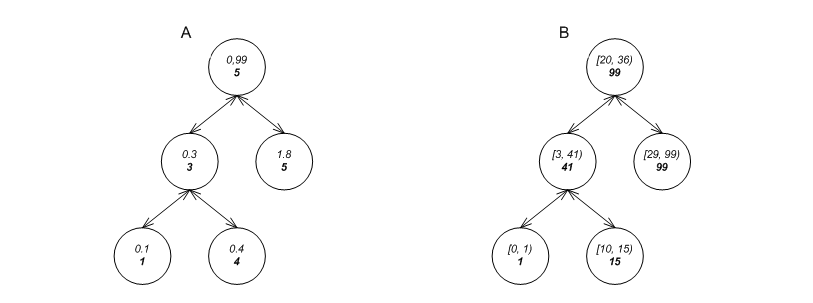Supporting such trees is difficult for a number of reasons:

1. There must be a way to specify what a node's metadata should be (if any).

2. Various operations can invalidate node invariants. The graphic below shows how a right rotation, performed on A, results in B, with nodes x and y having corrupted invariants (the grayed nodes in C). The graphic shows how an insert, performed on D, results in E, with nodes x and y having corrupted invariants (the grayed nodes in F). It is not feasible to know outside the tree the effect of an operation on the nodes of the tree.

3. The search paths of standard associative containers are defined by comparisons between keys, and not through metadata.

4. It is not feasible to know in advance which methods trees can support. Besides the usual `find` method, the first tree can support a `find_by_order` method, while the second can support an `overlaps` method.

Figure 22.23. Tree node invalidation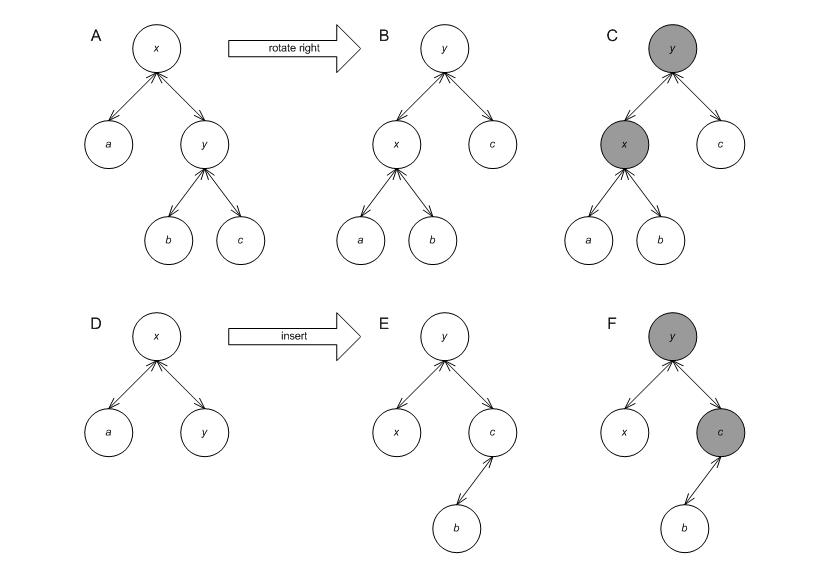These problems are solved by a combination of two means: node iterators, and template-template node updater parameters.

###### Node Iterators

Each tree-based container defines two additional iterator types, `const_node_iterator` and `node_iterator`. These iterators allow descending from a node to one of its children. Node iterator allow search paths different than those determined by the comparison functor. The `tree` supports the methods:

```	      const_node_iterator
node_begin() const;

node_iterator
node_begin();

const_node_iterator
node_end() const;

node_iterator
node_end();
```

The first pairs return node iterators corresponding to the root node of the tree; the latter pair returns node iterators corresponding to a just-after-leaf node.

###### Node Updator

The tree-based containers are parametrized by a `Node_Update` template-template parameter. A tree-based container instantiates `Node_Update` to some `node_update` class, and publicly subclasses `node_update`. The graphic below shows this scheme, as well as some predefined policies (which are explained below).

Figure 22.24. A tree and its update policy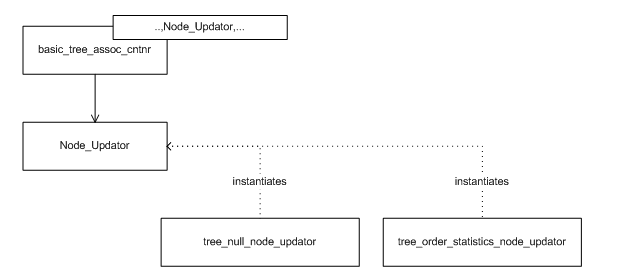`node_update` (an instantiation of `Node_Update`) must define `metadata_type` as the type of metadata it requires. For order statistics, e.g., `metadata_type` might be `size_t`. The tree defines within each node a `metadata_type` object.

`node_update` must also define the following method for restoring node invariants:

```	      void
operator()(node_iterator nd_it, const_node_iterator end_nd_it)
```

In this method, `nd_it` is a `node_iterator` corresponding to a node whose A) all descendants have valid invariants, and B) its own invariants might be violated; `end_nd_it` is a `const_node_iterator` corresponding to a just-after-leaf node. This method should correct the node invariants of the node pointed to by `nd_it`. For example, say node x in the graphic below label A has an invalid invariant, but its' children, y and z have valid invariants. After the invocation, all three nodes should have valid invariants, as in label B.

Figure 22.25. Restoring node invariants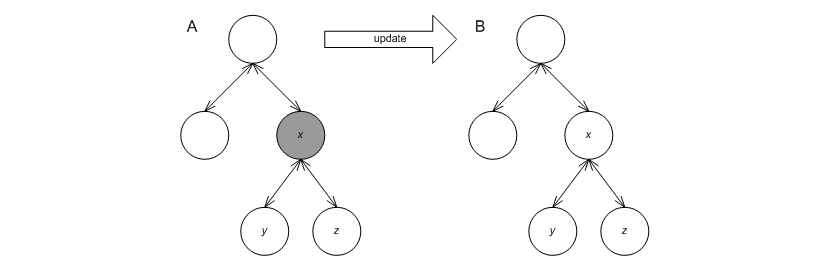When a tree operation might invalidate some node invariant, it invokes this method in its `node_update` base to restore the invariant. For example, the graphic below shows an `insert` operation (point A); the tree performs some operations, and calls the update functor three times (points B, C, and D). (It is well known that any `insert`, `erase`, `split` or `join`, can restore all node invariants by a small number of node invariant updates ([biblio.clrs2001]) .

Figure 22.26. Insert update sequence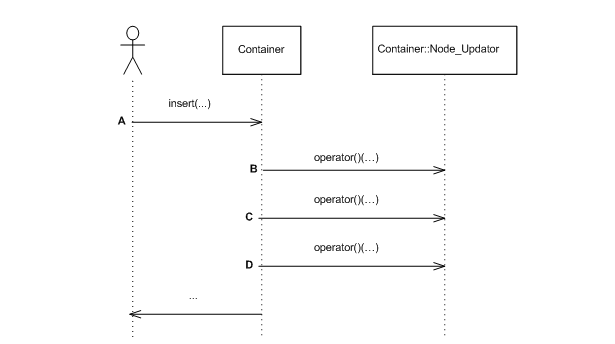To complete the description of the scheme, three questions need to be answered:

1. How can a tree which supports order statistics define a method such as `find_by_order`?

2. How can the node updater base access methods of the tree?

3. How can the following cyclic dependency be resolved? `node_update` is a base class of the tree, yet it uses node iterators defined in the tree (its child).

The first two questions are answered by the fact that `node_update` (an instantiation of `Node_Update`) is a public base class of the tree. Consequently:

1. Any public methods of `node_update` are automatically methods of the tree ([biblio.alexandrescu01modern]). Thus an order-statistics node updater, `tree_order_statistics_node_update` defines the `find_by_order` method; any tree instantiated by this policy consequently supports this method as well.

2. In C++, if a base class declares a method as `virtual`, it is `virtual` in its subclasses. If `node_update` needs to access one of the tree's methods, say the member function `end`, it simply declares that method as `virtual` abstract.

The cyclic dependency is solved through template-template parameters. `Node_Update` is parametrized by the tree's node iterators, its comparison functor, and its allocator type. Thus, instantiations of `Node_Update` have all information required.

This library assumes that constructing a metadata object and modifying it are exception free. Suppose that during some method, say `insert`, a metadata-related operation (e.g., changing the value of a metadata) throws an exception. Ack! Rolling back the method is unusually complex.

Previously, a distinction was made between redundant policies and null policies. Node invariants show a case where null policies are required.

Assume a regular tree is required, one which need not support order statistics or interval overlap queries. Seemingly, in this case a redundant policy - a policy which doesn't affect nodes' contents would suffice. This, would lead to the following drawbacks:

1. Each node would carry a useless metadata object, wasting space.

2. The tree cannot know if its `Node_Update` policy actually modifies a node's metadata (this is halting reducible). In the graphic below, assume the shaded node is inserted. The tree would have to traverse the useless path shown to the root, applying redundant updates all the way.

Figure 22.27. Useless update path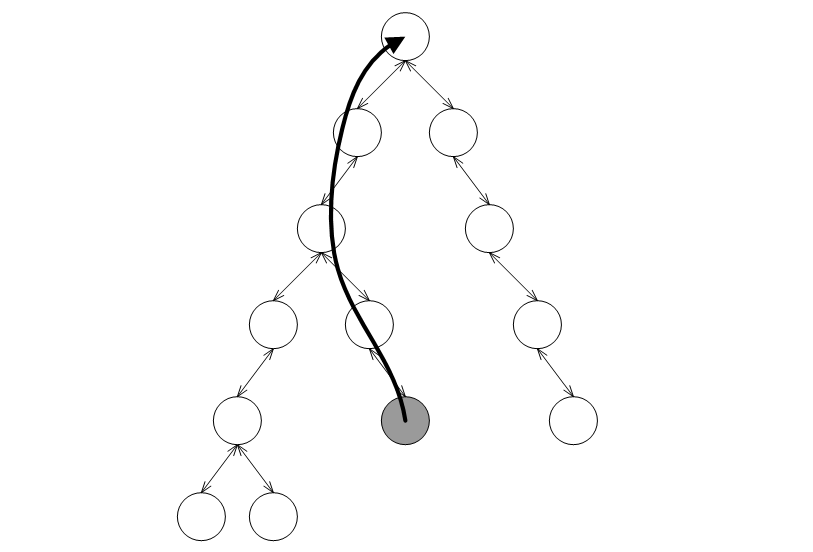A null policy class, `null_node_update` solves both these problems. The tree detects that node invariants are irrelevant, and defines all accordingly.

###### Split and Join

Tree-based containers support split and join methods. It is possible to split a tree so that it passes all nodes with keys larger than a given key to a different tree. These methods have the following advantages over the alternative of externally inserting to the destination tree and erasing from the source tree:

1. These methods are efficient - red-black trees are split and joined in poly-logarithmic complexity; ordered-vector trees are split and joined at linear complexity. The alternatives have super-linear complexity.

2. Aside from orders of growth, these operations perform few allocations and de-allocations. For red-black trees, allocations are not performed, and the methods are exception-free.

#### Trie

##### Interface

The trie-based container has the following declaration:

```	  template<typename Key,
typename Mapped,
typename Cmp_Fn = std::less<Key>,
typename Tag = pat_trie_tag,
template<typename Const_Node_Iterator,
typename Node_Iterator,
typename E_Access_Traits_,
typename Allocator_>
class Node_Update = null_node_update,
typename Allocator = std::allocator<char> >
class trie;
```

The parameters have the following meaning:

1. `Key` is the key type.

2. `Mapped` is the mapped-policy.

3. `E_Access_Traits` is described in below.

4. `Tag` specifies which underlying data structure to use, and is described shortly.

5. `Node_Update` is a policy for updating node invariants. This is described below.

6. `Allocator` is an allocator type.

The `Tag` parameter specifies which underlying data structure to use. Instantiating it by `pat_trie_tag`, specifies an underlying PATRICIA trie (explained shortly); any other tag is currently illegal.

Following is a description of a (PATRICIA) trie (this implementation follows [biblio.okasaki98mereable] and [biblio.filliatre2000ptset]).

A (PATRICIA) trie is similar to a tree, but with the following differences:

1. It explicitly views keys as a sequence of elements. E.g., a trie can view a string as a sequence of characters; a trie can view a number as a sequence of bits.

2. It is not (necessarily) binary. Each node has fan-out n + 1, where n is the number of distinct elements.

3. It stores values only at leaf nodes.

4. Internal nodes have the properties that A) each has at least two children, and B) each shares the same prefix with any of its descendant.

A (PATRICIA) trie has some useful properties:

1. It can be configured to use large node fan-out, giving it very efficient find performance (albeit at insertion complexity and size).

2. It works well for common-prefix keys.

3. It can support efficiently queries such as which keys match a certain prefix. This is sometimes useful in file systems and routers, and for "type-ahead" aka predictive text matching on mobile devices.

##### Details
###### Element Access Traits

A trie inherently views its keys as sequences of elements. For example, a trie can view a string as a sequence of characters. A trie needs to map each of n elements to a number in {0, n - 1}. For example, a trie can map a character `c` to

`static_cast<size_t>(c)`

.

Seemingly, then, a trie can assume that its keys support (const) iterators, and that the `value_type` of this iterator can be cast to a `size_t`. There are several reasons, though, to decouple the mechanism by which the trie accesses its keys' elements from the trie:

1. In some cases, the numerical value of an element is inappropriate. Consider a trie storing DNA strings. It is logical to use a trie with a fan-out of 5 = 1 + |{'A', 'C', 'G', 'T'}|. This requires mapping 'T' to 3, though.

2. In some cases the keys' iterators are different than what is needed. For example, a trie can be used to search for common suffixes, by using strings' `reverse_iterator`. As another example, a trie mapping UNICODE strings would have a huge fan-out if each node would branch on a UNICODE character; instead, one can define an iterator iterating over 8-bit (or less) groups.

trie is, consequently, parametrized by `E_Access_Traits` - traits which instruct how to access sequences' elements. `string_trie_e_access_traits` is a traits class for strings. Each such traits define some types, like:

```	    typename E_Access_Traits::const_iterator
```

is a const iterator iterating over a key's elements. The traits class must also define methods for obtaining an iterator to the first and last element of a key.

The graphic below shows a (PATRICIA) trie resulting from inserting the words: "I wish that I could ever see a poem lovely as a trie" (which, unfortunately, does not rhyme).

The leaf nodes contain values; each internal node contains two `typename E_Access_Traits::const_iterator` objects, indicating the maximal common prefix of all keys in the sub-tree. For example, the shaded internal node roots a sub-tree with leafs "a" and "as". The maximal common prefix is "a". The internal node contains, consequently, to const iterators, one pointing to `'a'`, and the other to `'s'`.

Figure 22.28. A PATRICIA trie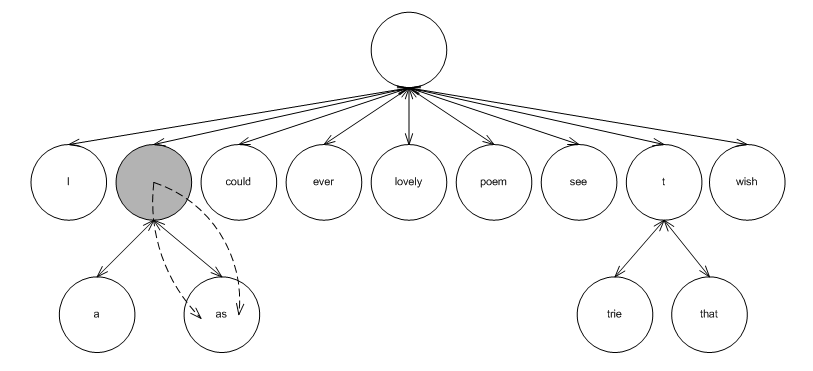###### Node Invariants

Trie-based containers support node invariants, as do tree-based containers. There are two minor differences, though, which, unfortunately, thwart sharing them sharing the same node-updating policies:

1. A trie's `Node_Update` template-template parameter is parametrized by `E_Access_Traits`, while a tree's `Node_Update` template-template parameter is parametrized by `Cmp_Fn`.

2. Tree-based containers store values in all nodes, while trie-based containers (at least in this implementation) store values in leafs.

The graphic below shows the scheme, as well as some predefined policies (which are explained below).

Figure 22.29. A trie and its update policy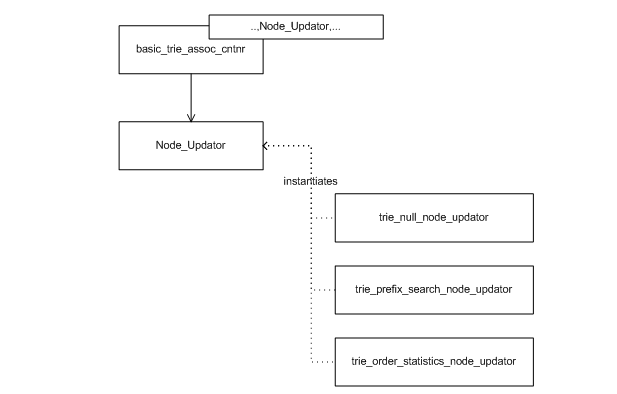This library offers the following pre-defined trie node updating policies:

1. `trie_order_statistics_node_update` supports order statistics.

2. `trie_prefix_search_node_update` supports searching for ranges that match a given prefix.

3. `null_node_update` is the null node updater.

###### Split and Join

Trie-based containers support split and join methods; the rationale is equal to that of tree-based containers supporting these methods.

#### List

##### Interface

The list-based container has the following declaration:

```	  template<typename Key,
typename Mapped,
typename Eq_Fn = std::equal_to<Key>,
typename Update_Policy = move_to_front_lu_policy<>,
typename Allocator = std::allocator<char> >
class list_update;
```

The parameters have the following meaning:

1. `Key` is the key type.

2. `Mapped` is the mapped-policy.

3. `Eq_Fn` is a key equivalence functor.

4. `Update_Policy` is a policy updating positions in the list based on access patterns. It is described in the following subsection.

5. `Allocator` is an allocator type.

A list-based associative container is a container that stores elements in a linked-list. It does not order the elements by any particular order related to the keys. List-based containers are primarily useful for creating "multimaps". In fact, list-based containers are designed in this library expressly for this purpose.

List-based containers might also be useful for some rare cases, where a key is encapsulated to the extent that only key-equivalence can be tested. Hash-based containers need to know how to transform a key into a size type, and tree-based containers need to know if some key is larger than another. List-based associative containers, conversely, only need to know if two keys are equivalent.

Since a list-based associative container does not order elements by keys, is it possible to order the list in some useful manner? Remarkably, many on-line competitive algorithms exist for reordering lists to reflect access prediction. (See [biblio.motwani95random] and [biblio.andrew04mtf]).

##### Details

###### Underlying Data Structure

The graphic below shows a simple list of integer keys. If we search for the integer 6, we are paying an overhead: the link with key 6 is only the fifth link; if it were the first link, it could be accessed faster.

Figure 22.30. A simple listList-update algorithms reorder lists as elements are accessed. They try to determine, by the access history, which keys to move to the front of the list. Some of these algorithms require adding some metadata alongside each entry.

For example, in the graphic below label A shows the counter algorithm. Each node contains both a key and a count metadata (shown in bold). When an element is accessed (e.g. 6) its count is incremented, as shown in label B. If the count reaches some predetermined value, say 10, as shown in label C, the count is set to 0 and the node is moved to the front of the list, as in label D.

Figure 22.31. The counter algorithm###### Policies

this library allows instantiating lists with policies implementing any algorithm moving nodes to the front of the list (policies implementing algorithms interchanging nodes are unsupported).

Associative containers based on lists are parametrized by a `Update_Policy` parameter. This parameter defines the type of metadata each node contains, how to create the metadata, and how to decide, using this metadata, whether to move a node to the front of the list. A list-based associative container object derives (publicly) from its update policy.

An instantiation of `Update_Policy` must define internally `update_metadata` as the metadata it requires. Internally, each node of the list contains, besides the usual key and data, an instance of ```typename Update_Policy::update_metadata```.

An instantiation of `Update_Policy` must define internally two operators:

```	    update_metadata
operator()();

bool
```

The first is called by the container object, when creating a new node, to create the node's metadata. The second is called by the container object, when a node is accessed ( when a find operation's key is equivalent to the key of the node), to determine whether to move the node to the front of the list.

The library contains two predefined implementations of list-update policies. The first is `lu_counter_policy`, which implements the counter algorithm described above. The second is `lu_move_to_front_policy`, which unconditionally move an accessed element to the front of the list. The latter type is very useful in this library, since there is no need to associate metadata with each element. (See [biblio.andrew04mtf]

###### Use in Multimaps

In this library, there are no equivalents for the standard's multimaps and multisets; instead one uses an associative container mapping primary keys to secondary keys.

List-based containers are especially useful as associative containers for secondary keys. In fact, they are implemented here expressly for this purpose.

To begin with, these containers use very little per-entry structure memory overhead, since they can be implemented as singly-linked lists. (Arrays use even lower per-entry memory overhead, but they are less flexible in moving around entries, and have weaker invalidation guarantees).

More importantly, though, list-based containers use very little per-container memory overhead. The memory overhead of an empty list-based container is practically that of a pointer. This is important for when they are used as secondary associative-containers in situations where the average ratio of secondary keys to primary keys is low (or even 1).

In order to reduce the per-container memory overhead as much as possible, they are implemented as closely as possible to singly-linked lists.

1. List-based containers do not store internally the number of values that they hold. This means that their `size` method has linear complexity (just like `std::list`). Note that finding the number of equivalent-key values in a standard multimap also has linear complexity (because it must be done, via `std::distance` of the multimap's `equal_range` method), but usually with higher constants.

2. Most associative-container objects each hold a policy object (a hash-based container object holds a hash functor). List-based containers, conversely, only have class-wide policy objects.

#### Priority Queue

##### Interface

The priority queue container has the following declaration:

```	  template<typename  Value_Type,
typename  Cmp_Fn = std::less<Value_Type>,
typename  Tag = pairing_heap_tag,
typename  Allocator = std::allocator<char > >
class priority_queue;
```

The parameters have the following meaning:

1. `Value_Type` is the value type.

2. `Cmp_Fn` is a value comparison functor

3. `Tag` specifies which underlying data structure to use.

4. `Allocator` is an allocator type.

The `Tag` parameter specifies which underlying data structure to use. Instantiating it by`pairing_heap_tag`,`binary_heap_tag`, `binomial_heap_tag`, `rc_binomial_heap_tag`, or `thin_heap_tag`, specifies, respectively, an underlying pairing heap ([biblio.fredman86pairing]), binary heap ([biblio.clrs2001]), binomial heap ([biblio.clrs2001]), a binomial heap with a redundant binary counter ([biblio.maverick_lowerbounds]), or a thin heap ([biblio.kt99fat_heaps]).

As mentioned in the tutorial, `__gnu_pbds::priority_queue` shares most of the same interface with `std::priority_queue`. E.g. if `q` is a priority queue of type `Q`, then `q.top()` will return the "largest" value in the container (according to ```typename Q::cmp_fn```). `__gnu_pbds::priority_queue` has a larger (and very slightly different) interface than `std::priority_queue`, however, since typically `push` and `pop` are deemed insufficient for manipulating priority-queues.

Different settings require different priority-queue implementations which are described in later; see traits discusses ways to differentiate between the different traits of different implementations.

##### Details
###### Iterators

There are many different underlying-data structures for implementing priority queues. Unfortunately, most such structures are oriented towards making `push` and `top` efficient, and consequently don't allow efficient access of other elements: for instance, they cannot support an efficient `find` method. In the use case where it is important to both access and "do something with" an arbitrary value, one would be out of luck. For example, many graph algorithms require modifying a value (typically increasing it in the sense of the priority queue's comparison functor).

In order to access and manipulate an arbitrary value in a priority queue, one needs to reference the internals of the priority queue from some form of an associative container - this is unavoidable. Of course, in order to maintain the encapsulation of the priority queue, this needs to be done in a way that minimizes exposure to implementation internals.

In this library the priority queue's `insert` method returns an iterator, which if valid can be used for subsequent `modify` and `erase` operations. This both preserves the priority queue's encapsulation, and allows accessing arbitrary values (since the returned iterators from the `push` operation can be stored in some form of associative container).

Priority queues' iterators present a problem regarding their invalidation guarantees. One assumes that calling `operator++` on an iterator will associate it with the "next" value. Priority-queues are self-organizing: each operation changes what the "next" value means. Consequently, it does not make sense that `push` will return an iterator that can be incremented - this can have no possible use. Also, as in the case of hash-based containers, it is awkward to define if a subsequent `push` operation invalidates a prior returned iterator: it invalidates it in the sense that its "next" value is not related to what it previously considered to be its "next" value. However, it might not invalidate it, in the sense that it can be de-referenced and used for `modify` and `erase` operations.

Similarly to the case of the other unordered associative containers, this library uses a distinction between point-type and range type iterators. A priority queue's `iterator` can always be converted to a `point_iterator`, and a `const_iterator` can always be converted to a `point_const_iterator`.

The following snippet demonstrates manipulating an arbitrary value:

```	    // A priority queue of integers.
priority_queue<int > p;

// Insert some values into the priority queue.
priority_queue<int >::point_iterator it = p.push(0);

p.push(1);
p.push(2);

// Now modify a value.
p.modify(it, 3);

assert(p.top() == 3);
```

It should be noted that an alternative design could embed an associative container in a priority queue. Could, but most probably should not. To begin with, it should be noted that one could always encapsulate a priority queue and an associative container mapping values to priority queue iterators with no performance loss. One cannot, however, "un-encapsulate" a priority queue embedding an associative container, which might lead to performance loss. Assume, that one needs to associate each value with some data unrelated to priority queues. Then using this library's design, one could use an associative container mapping each value to a pair consisting of this data and a priority queue's iterator. Using the embedded method would need to use two associative containers. Similar problems might arise in cases where a value can reside simultaneously in many priority queues.

###### Underlying Data Structure

There are three main implementations of priority queues: the first employs a binary heap, typically one which uses a sequence; the second uses a tree (or forest of trees), which is typically less structured than an associative container's tree; the third simply uses an associative container. These are shown in the graphic below, in labels A1 and A2, label B, and label C.

Figure 22.32. Underlying Priority-Queue Data-Structures.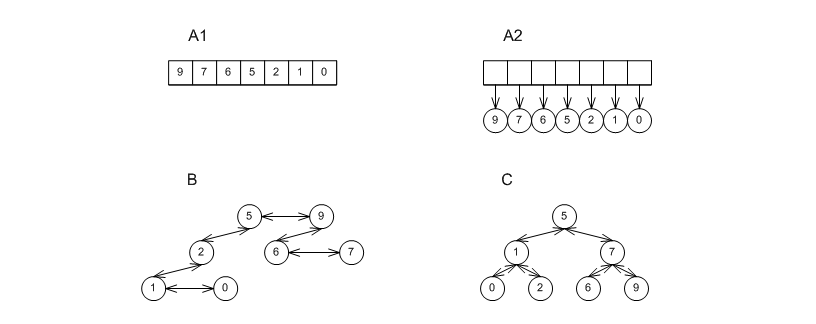Roughly speaking, any value that is both pushed and popped from a priority queue must incur a logarithmic expense (in the amortized sense). Any priority queue implementation that would avoid this, would violate known bounds on comparison-based sorting (see [biblio.clrs2001] and [biblio.brodal96priority]).

Most implementations do not differ in the asymptotic amortized complexity of `push` and `pop` operations, but they differ in the constants involved, in the complexity of other operations (e.g., `modify`), and in the worst-case complexity of single operations. In general, the more "structured" an implementation (i.e., the more internal invariants it possesses) - the higher its amortized complexity of `push` and `pop` operations.

This library implements different algorithms using a single class: `priority_queue`. Instantiating the `Tag` template parameter, "selects" the implementation:

1. Instantiating `Tag = binary_heap_tag` creates a binary heap of the form in represented in the graphic with labels A1 or A2. The former is internally selected by priority_queue if `Value_Type` is instantiated by a primitive type (e.g., an int); the latter is internally selected for all other types (e.g., `std::string`). This implementations is relatively unstructured, and so has good `push` and `pop` performance; it is the "best-in-kind" for primitive types, e.g., ints. Conversely, it has high worst-case performance, and can support only linear-time `modify` and `erase` operations.

2. Instantiating ```Tag = pairing_heap_tag``` creates a pairing heap of the form in represented by label B in the graphic above. This implementations too is relatively unstructured, and so has good `push` and `pop` performance; it is the "best-in-kind" for non-primitive types, e.g., `std:string`s. It also has very good worst-case `push` and `join` performance (O(1)), but has high worst-case `pop` complexity.

3. Instantiating ```Tag = binomial_heap_tag``` creates a binomial heap of the form repsented by label B in the graphic above. This implementations is more structured than a pairing heap, and so has worse `push` and `pop` performance. Conversely, it has sub-linear worst-case bounds for `pop`, e.g., and so it might be preferred in cases where responsiveness is important.

4. Instantiating ```Tag = rc_binomial_heap_tag``` creates a binomial heap of the form represented in label B above, accompanied by a redundant counter which governs the trees. This implementations is therefore more structured than a binomial heap, and so has worse `push` and `pop` performance. Conversely, it guarantees O(1) `push` complexity, and so it might be preferred in cases where the responsiveness of a binomial heap is insufficient.

5. Instantiating ```Tag = thin_heap_tag``` creates a thin heap of the form represented by the label B in the graphic above. This implementations too is more structured than a pairing heap, and so has worse `push` and `pop` performance. Conversely, it has better worst-case and identical amortized complexities than a Fibonacci heap, and so might be more appropriate for some graph algorithms.

Of course, one can use any order-preserving associative container as a priority queue, as in the graphic above label C, possibly by creating an adapter class over the associative container (much as `std::priority_queue` can adapt `std::vector`). This has the advantage that no cross-referencing is necessary at all; the priority queue itself is an associative container. Most associative containers are too structured to compete with priority queues in terms of `push` and `pop` performance.

###### Traits

It would be nice if all priority queues could share exactly the same behavior regardless of implementation. Sadly, this is not possible. Just one for instance is in join operations: joining two binary heaps might throw an exception (not corrupt any of the heaps on which it operates), but joining two pairing heaps is exception free.

Tags and traits are very useful for manipulating generic types. `__gnu_pbds::priority_queue` publicly defines `container_category` as one of the tags. Given any container `Cntnr`, the tag of the underlying data structure can be found via ```typename Cntnr::container_category```; this is one of the possible tags shown in the graphic below.

Figure 22.33. Priority-Queue Data-Structure Tags.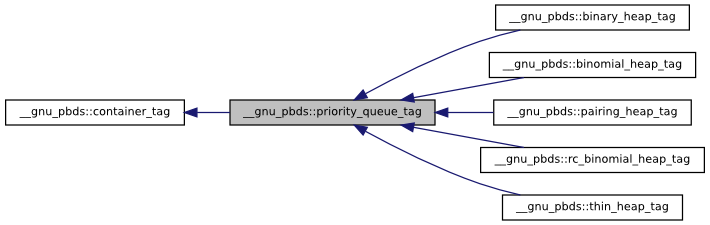Additionally, a traits mechanism can be used to query a container type for its attributes. Given any container `Cntnr`, then

`__gnu_pbds::container_traits<Cntnr>`

is a traits class identifying the properties of the container.

To find if a container might throw if two of its objects are joined, one can use

```	    container_traits<Cntnr>::split_join_can_throw
```

Different priority-queue implementations have different invalidation guarantees. This is especially important, since there is no way to access an arbitrary value of priority queues except for iterators. Similarly to associative containers, one can use

```	      container_traits<Cntnr>::invalidation_guarantee
```

to get the invalidation guarantee type of a priority queue.

It is easy to understand from the graphic above, what `container_traits<Cntnr>::invalidation_guarantee` will be for different implementations. All implementations of type represented by label B have `point_invalidation_guarantee`: the container can freely internally reorganize the nodes - range-type iterators are invalidated, but point-type iterators are always valid. Implementations of type represented by labels A1 and A2 have `basic_invalidation_guarantee`: the container can freely internally reallocate the array - both point-type and range-type iterators might be invalidated.

This has major implications, and constitutes a good reason to avoid using binary heaps. A binary heap can perform `modify` or `erase` efficiently given a valid point-type iterator. However, in order to supply it with a valid point-type iterator, one needs to iterate (linearly) over all values, then supply the relevant iterator (recall that a range-type iterator can always be converted to a point-type iterator). This means that if the number of `modify` or `erase` operations is non-negligible (say super-logarithmic in the total sequence of operations) - binary heaps will perform badly.